## Solution to 1986 Problem 43

 The equations of motion are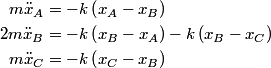\begin{align*}m\ddot{x}_A &= -k \left(x_A - x_B\right) \\2m\ddot{x}_B &= -k \left(x_B - x_A \right) - k \left(x_B - x...The normal mode frequencies are found by setting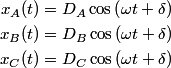\begin{align*}x_A(t) &= D_A\cos \left(\omega t + \delta \right) \\x_B(t) &= D_B\cos \left(\omega t + \delta \right) \...which gives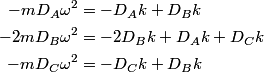\begin{align*}-m D_A\omega^2 &= -D_A k + D_B k \\-2mD_B\omega^2 &= -2 D_B k + D_A k + D_C k \\-mD_C\omega^2 &= - ...which can be written in matrix form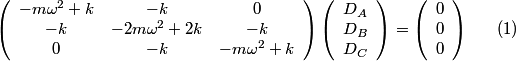\begin{align}\left(\begin{array}{ccc}- m \omega^2 + k & -k & 0 \\-k & - 2 m \omega^2 + 2k & -k\\0 & -k &a...There is a non-trivial solution for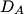$D_A$,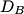$D_B$, and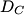$D_C$ if and only if the matrix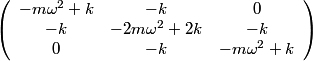\begin{align*}\left(\begin{array}{ccc}- m \omega^2 + k & -k & 0 \\-k & - 2 m \omega^2 + 2k & -k\\0 & -k &...has zero determinant. Upon setting the determinant equal to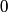$0$ and solving the resulting equation for$\omega$, one will presumably find that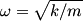$\omega = \sqrt{k/m}$ is a solution. Plug$\omega = \sqrt{k/m}$ into equation (16) and solve for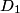$D_1$,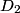$D_2$, and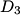$D_3$. This leads to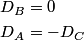\begin{align*}D_B &= 0 \\D_A &= - D_C\end{align*}which means that the motion corresponding to this normal mode is given by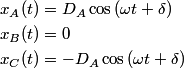\begin{align*}x_A(t) &= D_A\cos \left(\omega t + \delta \right) \\x_B(t) &= 0 \\x_C(t) &= -D_A\cos \left(\omega t...The statement that describes this motion is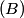$(B)$. Therefore, answer (B) is correct.